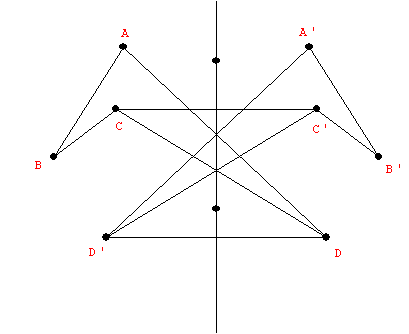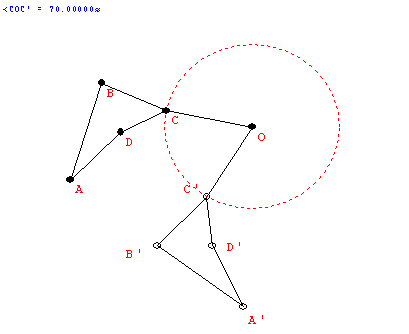# Tuesday  February 7

Cutting and reassembling polygons.

Convex:    Any two points in the figure have a line segment connecting them. If that line segment is always  inside the figure, then the figure is called "convex".

Making Dissection Puzzles [more links] :

Dissection of the plane--- Tilings of the plane:
Tessellations

• Activity: Tile plane with playing cards.
• FAPP watch Video: Possible tiles and tilings.
• One Regular Convex Polygon.
• Two or more Regular Convex Polygons.
• Other Polygon Tilings.
• Non polygonal tilings.

• One polygonal Tile: Quadrilateral Activity.
• An on-line tool for making tilings of the plane
• Regular and semiregular Tilings of the plane. Some explorations - classification.
• A tiling is a regular tiling if (i)it has a single tile shape that is a regular polygon and (ii) the vertices and edges of the tiles coincide (no overlapping edges)
• A tiling is a semi-regular tiling if (ii) each tile shape is a regular polygon, (ii) the vertices and edges of the tiles coincide (no overlapping edges) and (iii) every vertex has the same polygon types arranged around it.
• Naming tilings (Math Forum)
• The numbers represent the number of sides in the poygons.
• The order indcates the order in which the poygons are arranged about a vertex.
• Semiregular Tilings: global results!
Look at the results using wingeometry.
• Student lesson (Math Forum) a place for further explorations on-line.

• Symmetry Ideas
• Reflective symmetry: BI LATERAL SYMMETRY
T  C  O   0    I   A
• Folding line: "axis of symmetry"
• The "flip."
• The "mirror."
•• R(P) = P'  : A Transformation. Before: P .... After : P'
• If P is on the line (axis), then R(P)=P. "P remains fixed by the reflection."
• If P is not on the axis, then the line PP' is perpendicular to the axis and if Q is the point of intersection of PP' with the axis then m(PQ) = m(p'Q).

• Definition: We say F has a reflective symmetry wrt a line l if  there is a reflection  R about the line l where  R(P)=P' is still an element of F for every P in F....i.e.. R (F) = F. l is called the axis of symmetry.
• Examples of reflective symmetry:
Squares...  People

• Rotational Symmetry.
• Center of rotation. "rotational pole" (usually O) and angle/direction of rotation.
• The "spin."
•• R(P) = P' : A transformation
• If O is the center then R(O) = O.
• If the angle is 360 then R(P) = P for all P.... called the identity transformation.
• If  the angle is between 0 and 360 then only the center remains fixed.
• For any point P the angle POP'  is the same.
• Examples of rotational symmetry.

•  Now... what about finding all the reflective and rotational symmetries of a single figure?

• Symmetries of playing card.... classify the cards by having same symmetries.

Notice symmetry of clubs, diamonds, hearts, spades